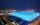# Volume and area

What is the volume of a cube which has an area of 361 cm2?

V =  466.6958 cm3

### Step-by-step explanation:Did you find an error or inaccuracy? Feel free to write us. Thank you!Tips to related online calculators
Tip: Our volume units converter will help you with the conversion of volume units.

## Related math problems and questions:

• Cube surfce2volumeCalculate the volume of the cube if its surface is 150 cm2.
• The cubeThe cube has a surface of 600 cm2. What is its volume?
• Cube surface and volumeThe surface of the cube is 500 cm2, how much cm3 will be its volume?
• The cubeThe cube has a surface area of 216 dm2. Calculate: a) the content of one wall, b) edge length, c) cube volume.
• Area to volumeIf the surface area of a cube is 486, find its volume.
• Cube 6The surface area of one wall cube is 1600 cm square. How many liters of water can fit into the cube?
• The cubeThe cube has a surface area of 486 m ^ 2. Calculate its volume.
• Cube 6Volume of the cube is 216 cm3, calculate its surface area.
• Cube surface and volumeFind the surface of the cube with a volume of 27 dm3.
• Cube wallThe surface of the first cube wall is 64 m2. The second cube area is 40% of the surface of the first cube. Determine the length of the edge of the second cube (x).
• Two rectangular boxesTwo rectangular boxes with dimensions of 5 cm, 8 cm, 10 cm, and 5 cm, 12 cm, 1 dm are to be replaced by a single cube box of the same cubic volume. Calculate its surface.
• Prism XThe prism with the edges of the lengths x cm, 2x cm, and 3x cm has volume 20250 cm3. What is the area of the surface of the prism?
• Cube edgeDetermine the edges of the cube when the surface is equal to 37.5 cm square.
• TerezaThe cube has an area of base 256 mm2. Calculate the edge length, volume, and area of its surface.
• Three cubesTwo cube-shaped boxes with edges a = 70 cm; b = 90 cm must be replaced by one cube-shaped box. What will be its edge?
• Cube 7Calculate the volume of a cube, whose sum of the lengths of all edges is 276 cm.
• Cuboid to cubeA cuboid with dimensions of 9 cm, 6 cm, and 4 cm has the same volume as a cube. Calculate the surface of this cube.